Bihar Board 12th Maths Objective Questions and Answers

## Bihar Board 12th Maths VVI Objective Questions Model Set 2 in English

Question 1.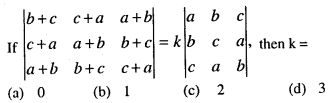(b) 1

Question 2.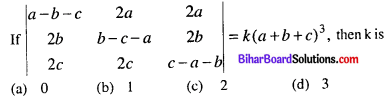(b) 1

Question 3.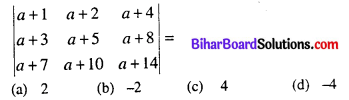(b) -2

Question 4.(c) -1

Question 5.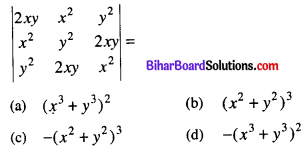(d) -(x3 + y3)2

Question 6.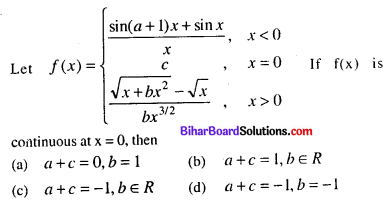(c) a + c = -1, b ∈ R

Question 7.(c) $$-\frac{1}{2}$$

Question 8.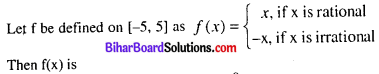(a) continuous at every x except x = 0
(b) discontinuous at every x except x = 0
(c) continuous everywhere
(d) discontinuous everywhere
(b) discontinuous at every x except x = 0

Question 9.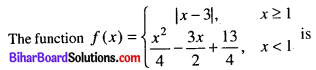(a) continuous at x = 1
(b) differentiable at x = 1
(c) continuous at x = -3
(d) All of these
(d) All of these

Question 10.
If $$f(x)=\frac{\sqrt{4+x}-2}{x}$$, x ≠ 0 be continuous at x = 0, then f(0) =
(a) $$\frac{1}{2}$$
(b) $$\frac{1}{4}$$
(c) 2
(d) $$\frac{3}{2}$$
(b) $$\frac{1}{4}$$

Question 11.
Moving along the x-axis there are two points with x = 10 + 6t, x = 3 + t2. The speed with which they are reaching from each other at the time of encounter is (x is an cm and t is in seconds)
(a) 16 cm/s
(b) 20 cm/s
(c) 8 cm/s
(d) 12 cm/s
(c) 8 cm/s

Question 12.
A particle is moving along the curve x = at2 + bt + c. If ac = b2, then particle would be moving with uniform
(a) rotation
(b) velocity
(c) acceleration
(d) retardation
(c) acceleration

Question 13.
The distance ‘s’ metres covered by a body in t seconds, is given by s = 3t2 – 8t + 5. The body will stop after
(a) 1 s
(b) $$\frac{3}{4}$$ s
(c) $$\frac{4}{3}$$ s
(d) 4 s
(c) $$\frac{4}{3}$$ s

Question 14.
The position of a point in time ‘t’ is given by x = a + bt – ct2, y = at + bt2. Its acceleration at time ‘t’ is
(a) b – c
(b) b + c
(c) 2b – 2c
(d) $$2 \sqrt{b^{2}+c^{2}}$$
(d) $$2 \sqrt{b^{2}+c^{2}}$$

Question 15.
The function f(x) = log (1 + x) – $$\frac{2 x}{2+x}$$
(a) (-1, ∞)
(b) (-∞, 0)
(c) (-∞, ∞)
(d) None of these
(a) (-1, ∞)

Question 16.(d) loge(10x + x10) + C

Question 17.(a) $$\frac{1}{(\log 2)^{3}} 2^{2^{2^{x}}}+C$$

Question 18.(b) $$-\frac{\cos ^{4} x}{4}+C$$

Question 19.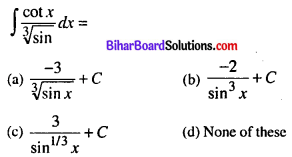(a) $$\frac{-3}{\sqrt{\sin x}}+C$$

Question 20.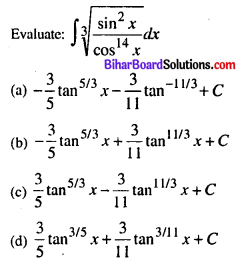(b) $$-\frac{3}{5} \tan ^{5 / 3} x+\frac{3}{11} \tan ^{11 / 3} x+C$$

Question 21.
The area bounded by the curve 2x2 + y2 = 2 is
(a) π sq. units
(b) √2 π sq. units
(c) $$\frac{\pi}{2}$$ sq. units
(d) 2π sq. units
(b) √2 π sq. units

Question 22.
Area of the ellipse $$\frac{x^{2}}{a^{2}}+\frac{y^{2}}{b^{2}}=1$$ is
(a) 4πab sq. units
(b) 2πab sq. units
(c) πab sq. units
(d) $$\frac{\pi a b}{2}$$ sq. units
(c) πab sq. units

Question 23.
Area of the region bounded by y = |x|, x ≤ 5 in the first quadrant is
(a) $$\frac{11}{2}$$ sq. units
(b) $$\frac{17}{2}$$ sq. units
(c) $$\frac{25}{2}$$ sq. units
(d) $$\frac{27}{2}$$ sq. units
(c) $$\frac{25}{2}$$ sq. units

Question 24.
Determine the area under the curve $$y=\sqrt{a^{2}-x^{2}}$$ included between the lines x = 0 and x = a.
(a) $$\frac{\pi a^{a}}{4}$$
(b) $$\frac{\pi a^{3}}{4}$$
(c) $$\frac{\pi a^{2}}{8}$$
(d) None of these
(a) $$\frac{\pi a^{a}}{4}$$

Question 25.
The area enclosed by curve $$\frac{x^{2}}{25}+\frac{y^{2}}{16}=1$$ is
(a) 10π sq. units
(b) 20π sq. units
(c) 5π sq. units
(d) 4π sq. units
(b) 20π sq. units

Question 26.
The order of the differential equation whose general solution is given by
y = (C1 + C2) cos(x + C3) – C4$$e^{x+C_{5}}$$
where C1, C2, C3, C4, C5 are arbitrary constant, is
(a) 5
(b) 4
(c) 3
(d) 2
(c) 3

Question 27.
The order of the differential equation whose general solution is given by y = (A + B) cos (x + C) + Dex is
(a) 4
(b) 3
(c) 2
(d) 1
(b) 3

Question 28.
Find the magnitude of vector $$3 \hat{i}+2 \hat{j}+12 \hat{k}$$
(a) √157
(b) 4√11
(c) √213
(d) 9√3
(a) √157

Direction (29) : Study the given parallelogram and answer the following questions.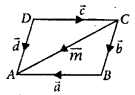Question 29.
Which of the following represents equal vectors?
(a) a, c
(b) b, d
(c) b, c
(d) m, d
(b) b, d

Question 30.
The cosines of the angle between any two diagonals of a cube is
(a) $$\frac{1}{3}$$
(b) $$\frac{1}{2}$$
(c) $$\frac{2}{3}$$
(d) $$\frac{1}{\sqrt{3}}$$
(a) $$\frac{1}{3}$$

Question 31.
Which of the following is false?
(a) 30°, 45°, 60° can be the direction angles of a line is space.
(b) 90°, 135°, 45° can be the direction angles of a line is space.
(c) 120°, 60°, 45° can be the direction angles of a line in space.
(d) 60°, 45°, 60° can be the direction angles of a line in space.
(a) 30°, 45°, 60° can be the direction angles of a line is space.

Question 32.
The optimal value of the objective function is attained at the points
(a) on X-axis
(b) on Y-axis
(c) which are corner points of the feasible region
(d) none of these
(c) which are corner points of the feasible region

Question 33.
Which one of the following is the order of the differential equation $$\frac{d^{2} y}{d x^{2}}+x^{3}\left(\frac{d y}{d x}\right)^{2}=x^{4}$$?
(a) 1
(b) 2
(c) 3
(d) 0
(b) 2

Question 34.
If two events A and B area such that P($$\bar{A}$$) = 0.3, P(b) = 0.4 and $$P(B | A \cup \bar{B})=$$
(a) $$\frac{1}{2}$$
(b) $$\frac{1}{3}$$
(c) $$\frac{2}{5}$$
(d) $$\frac{1}{4}$$
(d) $$\frac{1}{4}$$

Question 35.
If E and F are events such that 0 < P(F) < 1, then
(a) P(E|F) + P($$\bar{E}$$|F) = 1
(b) P(E|F) + P(E|$$\bar{F}$$) = 1
(c) P($$\bar{E}$$|F)+ P(E|$$\bar{F}$$) = 1
(d) P(E|$$\bar{F}$$) + P($$\bar{E}$$|F) = 0
(a) P(E|F) + P($$\bar{E}$$|F) = 1

Question 36.
Let R be a relation in N defined by R= {(1 + x, 1 + x2) : x ≤ 5, x ∈ N). Which of the following is false?
(a) R = {(2, 2), (3, 5), (4, 10), (5, 17), (6, 25)}
(b) Domain of R = (2, 3, 4, 5, 6)
(c) Range of R = {2, 5, 10, 17, 26}
(d) None of these
(a) R = {(2, 2), (3, 5), (4, 10), (5, 17), (6, 25)}

Question 37.
The relation R = {(1, 1), (2, 2), (3, 3), (1, 2), (2, 3), (1, 3)} on set A = {1, 2, 3} is
(a) Reflexive but not symmetric
(b) Reflexive but not transitive
(c) Symmetric and transitive
(d) Neither symmetric nor transitive
(a) Reflexive but not symmetric

Question 38.
Let P = {(x, y) | x2 + y2 = 1, x, y ∈ R}. Then, P is
(a) Reflexive
(b) Symmetric
(c) Transitive
(d) Anti-symmetric
(b) Symmetric

Question 39.
For real numbers x and y. we write xRy ⇔ x – y + √2.
(a) Reflexive
(b) Symmetric
(c) Transitive
(d) None of these
(a) Reflexive

Question 40.
Let L denote the set of all straight lines in a plane. Let a relation R be defined by αRβ ⇔ α ⊥ β, α, β ∈ L. Then, R is
(a) Reflexive only
(b) Symmetric only
(c) Transitive only
(d) None of these
(b) Symmetric only

Question 41.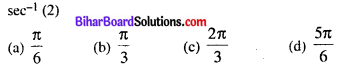(b) $$\frac{\pi}{3}$$

Question 42.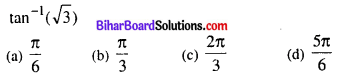(b) $$\frac{\pi}{3}$$

Question 43.(a) $$\frac{\pi}{4}$$

Question 44.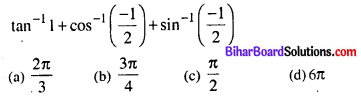(b) $$\frac{3 \pi}{4}$$

Question 45.(d) $$\frac{2 \pi}{3}$$

Question 46.
If $$\left[\begin{array}{ll} x+y & 2 x+z \\ x-y & 2 z+w \end{array}\right]=\left[\begin{array}{cc} 4 & 7 \\ 0 & 10 \end{array}\right]$$, then the values of x, y, z and w respectively are
(a) 2, 2, 3, 4
(b) 2, 3, 1, 2
(c) 3, 3, 0, 1
(d) None of these
(a) 2, 2, 3, 4

Question 47.
If $$\left[\begin{array}{cc} a+b & 2 \\ 5 & a b \end{array}\right]=\left[\begin{array}{cc} 6 & 2 \\ 5 & 8 \end{array}\right]$$, then find the values of a and b respectively
(a) 2, 4
(b) 4, 2
(c) Both (a) & (b)
(d) None of these
(c) Both (a) & (b)

Question 48.
For what values of x and y are the following matrices equal?(a) 2, 3
(b) 3, 4
(c) 2, 2
(d) 3, 3
(c) 2, 2

Question 49.
Find the values of a, b, c and d respectively if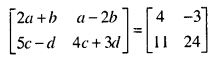(a) 1, 3, 9, 8
(b) 1, 2, 3, 4
(c) 1, 4, 8, 10
(d) 1, 5, 6, 7